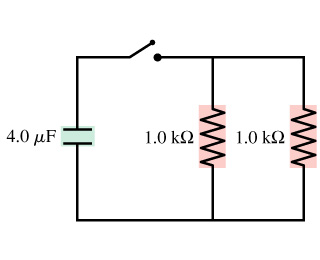# Problem: What is the time constant (units ms) for the discharge of the capacitor?

###### FREE Expert Solution

The time constant for an RC circuit:

$\overline{){\mathbf{\tau }}{\mathbf{=}}{\mathbf{R}}{\mathbf{C}}}$

Equivalent resistance for resistors in parallel:

$\overline{)\frac{\mathbf{1}}{{\mathbf{R}}_{\mathbf{eq}}}{\mathbf{=}}\frac{\mathbf{1}}{{\mathbf{R}}_{\mathbf{1}}}{\mathbf{+}}\frac{\mathbf{1}}{{\mathbf{R}}_{\mathbf{2}}}{\mathbf{+}}{\mathbf{.}}{\mathbf{.}}{\mathbf{.}}{\mathbf{+}}\frac{\mathbf{1}}{{\mathbf{R}}_{\mathbf{n}}}}$

or for 2 resistors:

$\overline{){{\mathbf{R}}}_{{\mathbf{eq}}}{\mathbf{=}}\frac{{\mathbf{R}}_{\mathbf{1}}{\mathbf{R}}_{\mathbf{2}}}{{\mathbf{R}}_{\mathbf{1}}\mathbf{+}{\mathbf{R}}_{\mathbf{2}}}}$

We are going to replace the two parallel resistors with an equivalent resistor then substitute in the equation for the time constant.

91% (303 ratings)###### Problem Details

What is the time constant (units ms) for the discharge of the capacitor?